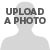# Maximo Open Forum

View Only

## Automation Script to Insert values in multiple rows#### Harshavardhan Kamepalli07-20-2021 03:46#### Venkatrao Y07-21-2021 09:38#### Harshavardhan Kamepalli07-30-2021 07:37#### Biplab Choudhury07-22-2021 08:44#### Harshavardhan Kamepalli07-30-2021 07:40• #### 1.  Automation Script to Insert values in multiple rows

Posted 07-20-2021 03:46
Hi All,
Hope you all are doing great in these hard times.
I have a requirement to insert a edited date value into a field on passing through a particular task node.
To achieve this, I have written a Action Launch point automation script and defined it in the workflow. It was working fine to this point. 'code' is the name of the variable defined.
``````from java.util import Calendar
from java.util import Date
from java.text import SimpleDateFormat
from psdi.server import MXServer
from psdi.mbo import Mbo

cal= Calendar.getInstance()
datetime=SimpleDateFormat().format(Date())
date=datetime.split(' ')
time=datetime.split(' ')

month=date.split('/')
day=date.split('/')
year=date.split('/')
hour=time.split(':')
min=time.split(':')

code='20'+year+'-'+month+'-'+day+'-'+hour+''+min	​``````

Sometimes there's gonna be multiple rows in which the value has to be inserted into multiple rows. I was trying to modify the script with While condition but it seems not working for me. The following is the script I was trying
``````from java.util import Calendar
from java.util import Date
from java.text import SimpleDateFormat
from psdi.server import MXServer
from psdi.mbo import Mbo

cal= Calendar.getInstance()
datetime=SimpleDateFormat().format(Date())
date=datetime.split(' ')
time=datetime.split(' ')

month=date.split('/')
day=date.split('/')
year=date.split('/')
hour=time.split(':')
min=time.split(':')

req=mbo.getMboSet("REQCONITEM")
i=0
records=req.getMbo(i)
while(records is not None):
i=i+1
code='20'+year+'-'+month+'-'+day+'-'+hour+''+min
records=req.getMbo(i)​``````

'REQCONITEM' is the name of the relationship
Any help on this one is appreciated.
#Customizations

------------------------------
Harshavardhan Kamepalli
Eidiko System Integrators
------------------------------

• #### 2.  RE: Automation Script to Insert values in multiple rows

Posted 07-21-2021 09:38
Hi,

You can use the moveFirst/moveNext methods in while loop. Or you can use 'for' loop as well.

###################################Using While Loop################################
``````from java.util import Calendar
from java.util import Date
from java.text import SimpleDateFormat
from psdi.server import MXServer
from psdi.mbo import Mbo

cal= Calendar.getInstance()
datetime=SimpleDateFormat().format(Date())
date=datetime.split(' ')
time=datetime.split(' ')

month=date.split('/')
day=date.split('/')
year=date.split('/')
hour=time.split(':')
min=time.split(':')​

req=mbo.getMboSet("REQCONITEM")
records=req.moveFirst()
while(records is not None):
code='20'+year+'-'+month+'-'+day+'-'+hour+''+min
records = req.moveNext()
``````

###################################Using For Loop################################

``````from java.util import Calendar
from java.util import Date
from java.text import SimpleDateFormat
from psdi.server import MXServer
from psdi.mbo import Mbo

cal= Calendar.getInstance()
datetime=SimpleDateFormat().format(Date())
date=datetime.split(' ')
time=datetime.split(' ')

month=date.split('/')
day=date.split('/')
year=date.split('/')
hour=time.split(':')
min=time.split(':')​

req=mbo.getMboSet("REQCONITEM")
records=req.count()
for i in range(0,records):
rowLevelMboRecords = req.getMbo(i)
code='20'+year+'-'+month+'-'+day+'-'+hour+''+min``````

------------------------------
Venkatrao Y
TCS
------------------------------

• #### 3.  RE: Automation Script to Insert values in multiple rows

Posted 07-30-2021 07:37
Hello Venkatrao,
Your code is not on point but helped to some extent. The following is the code that worked for me after making few changes:
``````from java.util import Calendar
from java.util import Date
from java.text import SimpleDateFormat
from psdi.server import MXServer
from psdi.mbo import Mbo

cal= Calendar.getInstance()
datetime=SimpleDateFormat().format(Date())
date=datetime.split(' ')
time=datetime.split(' ')

month=date.split('/')
day=date.split('/')
year=date.split('/')
hour=time.split(':')
min=time.split(':')

req=mbo.getMboSet("REQCONITEM")
req.reset()
records=req.moveFirst()
while(records is not None):
code='20'+year+'-'+month+'-'+day+'-'+hour+''+min
records.setValue('ISSUNCECODE',code)
records = req.moveNext()
req.save()​``````
Main thing was to use 'setValue' to insert value in each row in the loop and 'req.save()' function.

------------------------------
Harshavardhan Kamepalli
Eidiko System Integrators
------------------------------

• #### 4.  RE: Automation Script to Insert values in multiple rows

Posted 07-22-2021 08:44
Hi Harshavardhan,

How do you know the script is not working? Also, How are you using the code variable?
Your loop or single record is just setting the local variabel code but how are you using the code variable to know if it is working?

I would try to print the req mboset count in logs or in a variable to check if the relationship is working.
If the count is coming up as correct then please share the whole code.

------------------------------
Biplab Choudhury
Tata Consultancy Services
------------------------------

• #### 5.  RE: Automation Script to Insert values in multiple rows

Posted 07-30-2021 07:40
Hi Biplab,
Yes, I was using a variable, the attribute stored in code.
I was testing the code functionality by selecting multiple records to test the scenario.
The following code solves the problem.
``````from java.util import Calendar
from java.util import Date
from java.text import SimpleDateFormat
from psdi.server import MXServer
from psdi.mbo import Mbo

cal= Calendar.getInstance()
datetime=SimpleDateFormat().format(Date())
date=datetime.split(' ')
time=datetime.split(' ')

month=date.split('/')
day=date.split('/')
year=date.split('/')
hour=time.split(':')
min=time.split(':')

req=mbo.getMboSet("REQCONITEM")
req.reset()
records=req.moveFirst()
while(records is not None):
code='20'+year+'-'+month+'-'+day+'-'+hour+''+min
records.setValue('ISSUNCECODE',code)
records = req.moveNext()
req.save()​``````

------------------------------
Harshavardhan Kamepalli
Eidiko System Integrators
------------------------------

• #### 6.  RE: Automation Script to Insert values in multiple rows

Posted 07-22-2021 12:47
You might also try
# ============================
#iterable
from com.ibm.tivoli.maximo.util.mbo import IterableMboSet
for myMbo in IterableMboSet(myMboSet)
# ============================

I couldn't tell you the relative differences between either solution (perhaps some simplification)...

------------------------------
keith simmons
Aquitas Solutions
------------------------------